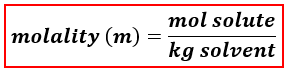# Problem: HCl density 1.19 g/cm3 and 37% solute by mass. Calculate the molality. Please explain step by step.

###### FREE Expert Solution

We’re being asked to calculate for the molality of a 37% by mass HCl solution.

When calculating for molality, we use the following equation:We will calculate the molality of the solution using the following steps:

Step 1: Calculate the mass and moles of solute

Step 2: Calculate the mass of the solvent (in kg).

Step 3: Calculate the molality of the solution.

Solute → HCl

Solvent → water (H2O)

83% (32 ratings)###### Problem Details

HCl density 1.19 g/cm3 and 37% solute by mass. Calculate the molality. Please explain step by step.